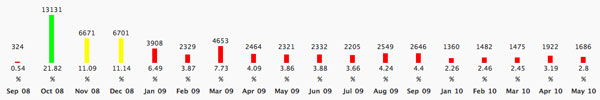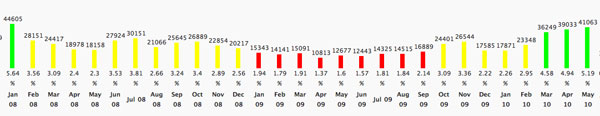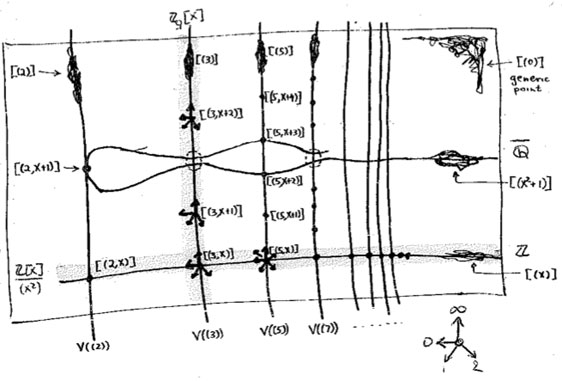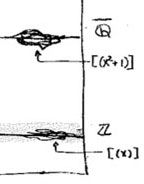# Tag: wordpress

As John Baez (and hopefully others) noticed most links here on NeB are now screwed up.

For years I’ve used the Markdown syntax to include links in posts (that is something like [this is what you see](and here is the URL)). NeB used an older version ( Version 1.0.1k) of php Markdown to convert these to proper links (as I did have problems with the newer version).

Ever since the Return to LaTeX post, I’m trying to re-educate myself to write posts in LaTeX and convert them into wp-format using the Latex2WP python script.

Unfortunately this script doesn’t go together nicely with the Markdown-syntax, forcing me to go over the converted latex-file almost line by line fixing errors in order to be able to post. Not the time-saver I had in mind…

I hope to post a lot of new material once the semester finishes and therefore disabled the Markdown-plugin. This causes all links to remain in Markdown-format, which is a nuisance but, if you really want to follow the link it is still there for you to copy-paste. Depending on how exciting the christmas-break will be I’ll try to hard-code the links in some of the more popular posts.

If someone knows of an elegant solution, such as a micro-markdown wp-plugin only parsing links, please drop a comment.

UPDATE : I have downloaded the Markdown quicktags plugin. It replaces the standard HTML-editor of WordPress with a Markdown-editor. There is an option “Render” which transforms Markdown to HTML and then updating the post will save the HTML-version. This looks like a relative quick way to get my old post-links back. So far, I’ve rescued the posts of the last year, more later. Hopefully, this causes no overload in your RSS-aggregator…

Via Tanya Khovanova I learned yesterday of the 50 best math blogs for math-majors list by OnlineDegree.net. Tanya’s blog got in 2nd (congrats!) and most of the blogs I sort of follow made it to the list : the n-category cafe (5), not even wrong (6), Gowers (12), Tao (13), good math bad math (14), rigorous trivialities (18), the secret blogging seminar (20), arcadian functor (28) (btw. Kea’s new blog is now at arcadian pseudofunctor), etc., etc. . Sincere congrats to you all!

NeverEndingBooks didn’t make it to the list, and I can live with that. For reasons only relevant to myself, posting has slowed down over the last year and the most recent post dates back from february!

More puzzling to me was the fact that F-un mathematics got in place 26! OnlineDegree had this to say about F-un Math : “Any students studying math must bookmark this blog, which provides readers with a broad selection of undergraduate and graduate concerns, quotes, research, webcasts, and much, much more.” Well, personally I wouldn’t bother to bookmark this site as prospects for upcoming posts are virtually inexistent…

As I am privy to both sites’ admin-pages, let me explain my confusion by comparing their monthly hits. Here’s the full F-un historyAfter a flurry of activity in the fall of 2008, both posting and attendance rates dropped, and presently the site gets roughly 50 hits-a-day. Compare this to the (partial) NeB historyThe whopping 45000 visits in january 2008 were (i think) deserved at the time as there was then a new post almost every other day. On the other hand, the green bars to the right are a mystery to me. It appears one is rewarded for not posting at all…

The only explanation I can offer is that perhaps more and more people are recovering from the late 2008-depression and do again enjoy reading blog-posts. Google then helps blogs having a larger archive (500 NeB-posts compared to about 20 genuine Fun-posts) to attract a larger audience, even though the blog is dormant.

But this still doesn’t explain why FunMath made it to the top 50-list and NeB did not. Perhaps the fault is entirely mine and a consequence of a bad choice of blog-title. ‘NeverEndingBooks’ does not ring like a math-blog, does it?

Still, I’m not going to change the title into something more math-related. NeverEndingBooks will be around for some time (unless my hard-disk breaks down). On the other hand, I plan to start something entirely new and learn from the mistakes I made over the past 6 years. Regulars of this blog will have a pretty good idea of the intended launch date, not?

Until then, my online activity will be limited to tweets.

To most mathematicians, a good LaTeX-frontend (such as TeXShop for Mac-users) is the crucial tool to get the work done. We use it to draft ideas, write papers and courses, or even to take notes during lectures.

However, after six years of blogging, my own LaTeX-routine became rusty. I rarely open a new tex-document, and when I do, I’d rather copy-paste the long preamble from an old file than to start from scratch with a minimal list of packages and definitions needed for the job at hand. The few times I put a paper on the arXiv, the resulting text resembles a blog-post more than a mathematical paper, here’s an example.

As I desperately need to get some math-writing done, I need to pull myself away from the lure of an ever-open WordPress admin browser-screen and reacquaint myself with the far more efficient LaTeX-environment.

Perhaps even my blogging will benefit from the change. Whereas I used to keep on adding to most of my tex-files in order to keep them up-to-date, I rarely edit a blog-post after hitting the ’publish’ button. If I really want to turn some of my better posts into a book, I need them in a format suitable for neverending polishing, without annoying the many RSS-feed aggregators out there.

Who better than Terry Tao to teach me a more proficient way of blogging? A few days ago, Terry announced he will soon have his 5th (!!) book out, after three years of blogging…

How does he manage to do this? Well, as far as I know, Terry blogs in LaTeX and then uses a python-script called LaTeX2WP ’a program that converts a LaTeX file into something that is ready to be cut and pasted into WordPress. This way, you can write, and preview, your post in LaTeX, then run LaTeX2WP, and post into WordPress whatever comes out.’ More importantly, one retains a pure-tex-file of the post on which one can keep on editing to get it into a (book)-publishable form, eventually.

Nice, but one can do even better, as Eric from Curious Reasoning worked out. He suggests to install two useful python-packages : WordPressLib “with this library you can control remotely a WordPress installation. Use of library is very simple, you can write a small scripts or full applications that allows you to automate publishing of articles on your blog/site powered by WordPress” and plasTeX “plasTeX is a LaTeX document processing framework written entirely in Python. It currently comes bundled with an XHTML renderer (including multiple themes), as well as a way to simply dump the document to a generic form of XML”. Installation is easy : download and extract the files somewhere, go there and issue a **sudo python setup.py install** to add the packages to your python.

Finally, get Eric’s own wplatex package and install it as explained there. WpLaTeX has all the features of LaTeX2WP and much more : one can add titles, tags and categories automatically and publish the post from the command-line without ever having to enter the taboo WordPress-admin page! Here’s what I’ve written by now in TeXShopI’ve added the screenshot and the script will know where to find it online for the blog-version as well as on my hard-disk for the tex-version. Very handy is the iftex … fi versus ifblog … fi alternative which allows you to add pure HTML to get the desired effect, when needed. Remains only to go into Terminal and issue the command

wplpost -x http://www.neverendingbooks.org/xmlrpc.php ReturnToLatex.tex

(if your blog is on WordPress.com it even suffices to give its name, rather than this work-around for stand-alone wordpress blogs). The script asks for my username and password and will convert the tex-file and post it automatic.

Ever since I’ve upgraded to Snow Leopard I’ve been having problems with the webserver.

At first there were the ‘obvious’ problems : mysql-connection lost and php-error message. These were swiftly dealt with using the excellent Snow Leopard, Apache, PHP, MySQL and WordPress! advice from ‘tady’.

Right now, access to this blog is extremely slow (and often impossible), certainly via the admin-page. The problem appears to be that most of my CPU is used by lots of pdfetex-processes owned by www. Hence the conjecture that it is a problem with either LaTeXRender or WP LaTeX.

Anyone experiencing a similar problem, or knowing a trick to resolve it? Takk.

A comment-thread well worth following while on vacation was Algebraic Geometry without Prime Ideals at the Secret Blogging Seminar. Peter Woit became lyric about it :

My nomination for the all-time highest quality discussion ever held in a blog comment section goes to the comments on this posting at Secret Blogging Seminar, where several of the best (relatively)-young algebraic geometers in the business discuss the foundations of the subject and how it should be taught.

I follow far too few comment-sections to make such a definite statement, but found the contributions by James Borger and David Ben-Zvi of exceptional high quality. They made a case for using Grothendieck’s ‘functor of points’ approach in teaching algebraic geometry instead of the ‘usual’ approach via prime spectra and their structure sheaves.

The text below was written on december 15th of last year, but never posted. As far as I recall it was meant to be part two of the ‘Brave New Geometries’-series starting with the Mumford’s treasure map post. Anyway, it may perhaps serve someone unfamiliar with Grothendieck’s functorial approach to make the first few timid steps in that directions.Allyn Jackson’s beautiful account of Grothendieck’s life “Comme Appele du Neant, part II” (the first part of the paper can be found here) contains this gem :

“One striking characteristic of Grothendieck’s
mode of thinking is that it seemed to rely so little
on examples. This can be seen in the legend of the
so-called “Grothendieck prime”.

In a mathematical
conversation, someone suggested to Grothendieck
that they should consider a particular prime number.
“You mean an actual number?” Grothendieck
asked. The other person replied, yes, an actual
prime number. Grothendieck suggested, “All right,
take 57.”

But Grothendieck must have known that 57 is not
prime, right? Absolutely not, said David Mumford
of Brown University. “He doesn’t think concretely.””

We have seen before how Mumford’s doodles allow us to depict all ‘points’ of the affine scheme $\mathbf{spec}(\mathbb{Z}[x])$, that is, all prime ideals of the integral polynomial ring $\mathbb{Z}[x]$.
Perhaps not too surprising, in view of the above story, Alexander Grothendieck pushed the view that one should consider all ideals, rather than just the primes. He achieved this by associating the ‘functor of points’ to an affine scheme.

Consider an arbitrary affine integral scheme $X$ with coordinate ring $\mathbb{Z}[X] = \mathbb{Z}[t_1,\ldots,t_n]/(f_1,\ldots,f_k)$, then any ringmorphism
$\phi~:~\mathbb{Z}[t_1,\ldots,t_n]/(f_1,\ldots,f_k) \rightarrow R$
is determined by an n-tuple of elements $~(r_1,\ldots,r_n) = (\phi(t_1),\ldots,\phi(t_n))$ from $R$ which must satisfy the polynomial relations $f_i(r_1,\ldots,r_n)=0$. Thus, Grothendieck argued, one can consider $~(r_1,\ldots,r_n)$ an an ‘$R$-point’ of $X$ and all such tuples form a set $h_X(R)$ called the set of $R$-points of $X$. But then we have a functor

$h_X~:~\mathbf{commutative rings} \rightarrow \mathbf{sets} \qquad R \mapsto h_X(R)=Rings(\mathbb{Z}[t_1,\ldots,t_n]/(f_1,\ldots,f_k),R)$

So, what is this mysterious functor in the special case of interest to us, that is when $X = \mathbf{spec}(\mathbb{Z}[x])$?
Well, in that case there are no relations to be satisfied so any ringmorphism $\mathbb{Z}[x] \rightarrow R$ is fully determined by the image of $x$ which can be any element $r \in R$. That is, $Ring(\mathbb{Z}[x],R) = R$ and therefore Grothendieck’s functor of points
$h_{\mathbf{spec}(\mathbb{Z}[x]}$ is nothing but the forgetful functor.

But, surely the forgetful functor cannot give us interesting extra information on Mumford’s drawing?
Well, have a look at the slightly extended drawing below :What are these ‘smudgy’ lines and ‘spiky’ points? Well, before we come to those let us consider the easier case of identifying the $R$-points in case $R$ is a domain. Then, for any $r \in R$, the inverse image of the zero prime ideal of $R$ under the ringmap $\phi_r~:~\mathbb{Z}[x] \rightarrow R$ must be a prime ideal of $\mathbb{Z}[x]$, that is, something visible in Mumford’s drawing. Let’s consider a few easy cases :For starters, what are the $\mathbb{Z}$-points of $\mathbf{spec}(\mathbb{Z}[x])$? Any natural number $n \in \mathbb{Z}$ determines the surjective ringmorphism $\phi_n~:~\mathbb{Z}[x] \rightarrow \mathbb{Z}$ identifying $\mathbb{Z}$ with the quotient $\mathbb{Z}[x]/(x-n)$, identifying the ‘arithmetic line’ $\mathbf{spec}(\mathbb{Z}) = { (2),(3),(5),\ldots,(p),\ldots, (0) }$ with the horizontal line in $\mathbf{spec}(\mathbb{Z}[x])$ corresponding to the principal ideal $~(x-n)$ (such as the indicated line $~(x)$).

When $\mathbb{Q}$ are the rational numbers, then $\lambda = \frac{m}{n}$ with $m,n$ coprime integers, in which case we have $\phi_{\lambda}^{-1}(0) = (nx-m)$, hence we get again an horizontal line in $\mathbf{spec}(\mathbb{Z}[x])$. For $\overline{\mathbb{Q}}$, the algebraic closure of $\mathbb{Q}$ we have for any $\lambda$ that $\phi_{\lambda}^{-1}(0) = (f(x))$ where $f(x)$ is a minimal integral polynomial for which $\lambda$ is a root.
But what happens when $K = \mathbb{C}$ and $\lambda$ is a trancendental number? Well, in that case the ringmorphism $\phi_{\lambda}~:~\mathbb{Z}[x] \rightarrow \mathbb{C}$ is injective and therefore $\phi_{\lambda}^{-1}(0) = (0)$ so we get the whole arithmetic plane!

In the case of a finite field $\mathbb{F}_{p^n}$ we have seen that there are ‘fat’ points in the arithmetic plane, corresponding to maximal ideals $~(p,f(x))$ (with $f(x)$ a polynomial of degree $n$ which remains irreducible over $\mathbb{F}_p$), having $\mathbb{F}_{p^n}$ as their residue field. But these are not the only $\mathbb{F}_{p^n}$-points. For, take any element $\lambda \in \mathbb{F}_{p^n}$, then the map $\phi_{\lambda}$ takes $\mathbb{Z}[x]$ to the subfield of $\mathbb{F}_{p^n}$ generated by $\lambda$. That is, the $\mathbb{F}_{p^n}$-points of $\mathbf{spec}(\mathbb{Z}[x])$ consists of all fat points with residue field $\mathbb{F}_{p^n}$, together with slightly slimmer points having as their residue field $\mathbb{F}_{p^m}$ where $m$ is a divisor of $n$. In all, there are precisely $p^n$ (that is, the number of elements of $\mathbb{F}_{p^n}$) such points, as could be expected.

Things become quickly more interesting when we consider $R$-points for rings containing nilpotent elements.